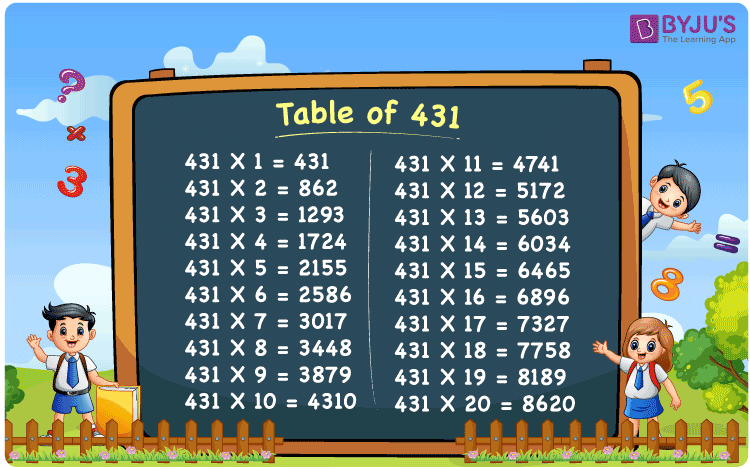Checkout JEE MAINS 2022 Question Paper Analysis : Checkout JEE MAINS 2022 Question Paper Analysis :

# Table of 431

The Table of 431, given at BYJU’S, mainly aims at improving the students skills, from counting numbers using fingers to mental calculation. The reason behind this is, most of the competitive exams provide less time to answer each question. If a student is thorough with the multiplication tables, scoring good marks in their final exams will be very easy. The multiplication table of 431 PDF can be downloaded for free by the students, by using the link given here.

## Table of 431 Chart## What is the 431 Times Table?

The multiplication table of 431 explains the process of multiplication and the pattern of multiples. The repeated addition and multiplication is mainly discussed here so that students will have a strong knowledge of these fundamental concepts during their higher education. Multiplication of numbers till 10 and the concept of addition is provided in the form of a chart for reference of the students.

 431 × 1 = 431 431 431 × 2 = 862 431 + 431 = 862 431 × 3 = 1293 431 + 431 + 431 = 1293 431 × 4 = 1724 431 + 431 + 431 + 431 = 1724 431 × 5 = 2155 431 + 431 + 431 + 431 + 431 = 2155 431 × 6 = 2586 431 + 431 + 431 + 431 + 431 + 431 = 2586 431 × 7 = 3017 431 + 431 + 431 + 431 + 431 + 431 + 431 = 3017 431 × 8 = 3448 431 + 431 + 431 + 431 + 431 + 431 + 431 + 431 = 3448 431 × 9 = 3879 431 + 431 + 431 + 431 + 431 + 431 + 431 + 431 + 431 = 3879 431 × 10 = 4310 431 + 431 + 431 + 431 + 431 + 431 + 431 + 431 + 431 + 431 = 4310

## Multiplication Table of 431

The Table of 431 is prepared by accomplished teachers at BYJU’S who possess vast knowledge in the respective subject. By revising the multiplication table of 431, students will be able to recall the answer for questions based on the multiples of 431. Below students can find the multiplication of 431 till number upto 20 in the form of a chart.

 431 × 1 = 431 431 × 2 = 862 431 × 3 = 1293 431 × 4 = 1724 431 × 5 = 2155 431 × 6 = 2586 431 × 7 = 3017 431 × 8 = 3448 431 × 9 = 3879 431 × 10 = 4310 431 × 11 = 4741 431 × 12 = 5172 431 × 13 = 5603 431 × 14 = 6034 431 × 15 = 6465 431 × 16 = 6896 431 × 17 = 7327 431 × 18 = 7758 431 × 19 = 8189 431 × 20 = 8620

## Solved Example on the Table of 431

Q.1: 4 houses were painted in a day. How many houses will be painted in 431 days?

Solution: Given,

Number of houses painted in a day = 4

In 431 days number of houses that will be painted = 431 x 4

= 1724

## Frequently Asked Questions on the Table of 431

### Evaluate 431 multiplied by 12.

Using the table of 431, 431 multiplied by 12 is 431 x 12 = 5172.

### Which number should be multiplied with 431 to obtain 5603?

In the table of 431, 431 x 13 = 4620. So, 13 should be multiplied with 431 to get 5603.

### Calculate 431 times 11 plus 4 minus 10.

From the table of 431, 431 times 11 plus 4 minus 10 can be written as 431 x (11 + 4 – 10) = 431 x 5 = 2155